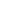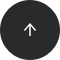Back to articles list- 10 minutes read

# Understanding Execution Plans in PostgreSQL

Execution plans can become a very useful tool for every database developer. They provide a deeper understanding of the mechanisms applied in the processing of queries. This article will take a closer look at how execution plans are retrieved and how to read them.

### What Are Execution Plans?

SQL is, to a great extent, a declarative language. The user defines what should be done but does not specify how the queries should be executed. There are many ways in which certain parts of an SQL statement can be processed. For example, predicates can be computed in any sequence and subqueries can be turned into joins if necessary.

The so-called query optimizer attempts to determine the most efficient way to execute a statement based on their estimated costs and creates an execution plan. This plan is later executed step-by-step by the database. Consequently, when you come across statements which execute slowly in your database, the execution plan should be the starting point for your analysis.

### Retrieving the Execution Plan

The execution plan of a statement can be retrieved using the keyword EXPLAIN in front of an SQL statement, as in the following example:

```EXPLAIN SELECT * FROM user;
```

As a result of the above statement, the system will print the execution plan:

```                        QUERY PLAN
----------------------------------------------------------
Seq Scan on users  (cost=0.00..5.24 rows=234 width=41)
```

In the above example, the generic form of EXPLAIN is used, which means that the statement is not executed and we only get a prediction of what is likely to happen. We can, however, make use of the ANALYZE keyword which does actually run the query and which prints more detailed information to the output, for example:

```EXPLAIN ANALYZE SELECT * FROM users;

QUERY PLAN
-----------------------------------------------------------
Seq Scan on users (cost=0.00..5.21 rows=173 width=118)
(actual time=0.018..0.018 rows=0 loops=1)
Total runtime: 0.020 ms
```

As we can see, an additional line appeared, explaining the actual time spent on the execution of the query as well as the number of rows retrieved.

`EXPLAIN` is most commonly run on `SELECT` statements, but it is possible to apply it to any `INSERT`, `UPDATE`, `DELETE`, `EXECUTE` or `DECLARE` clause. Keep in mind, however, that your statement will be executed when you use `ANALYZE`. This means that an `INSERT`, `UPDATE`, `DELETE` or `EXECUTE` clause will affect your data. To avoid this, you can wrap your statement in a transaction, for example:

```BEGIN;
EXPLAIN ANALYZE ...;
ROLLBACK;
```

### How to Read an Execution Plan?

The execution plan provides information about the specific operations chosen by the query optimizer. Let’s analyse some typical examples of its usage.

```=> EXPLAIN SELECT * FROM users;

QUERY PLAN
---------------------------------------------------------
Seq Scan on users (cost=0.00..5.24 rows=213 width=41)
```

The above result indicates that a full table scan (we will talk about it later) has been performed on the table users. Four numbers are then specified in the brackets with the following meaning:

• The two cost values specify the startup cost and the total cost, respectively. By default, the cost variables are measured with the cost of a sequential page fetch and the other cost variables are set with reference to that. The startup cost describes the amount of work expended before output scan can start. The total cost defines the estimated cost if all rows were to be retrieved (which is not always the case – if your SQL statement comprises a `LIMIT` clause, for example, the algorithm will stop after retrieving a certain amount of rows).
• The rows value estimates the number of rows output by the plan if executed to completion.
• The `width` value defines the estimated average width of rows in bytes.

The two vitally important decisions which the optimizer has to make are the choice of a scan method and the choice of a join method. In principle, PostgreSQL makes use of four different scan methods and three join methods.

### Scan Methods

Let’s first analyse the techniques used to perform scanning.

• Sequential Scan

Sequential Scan in PostgreSQL scans the entire table to choose the desired rows. This is the least effective scanning method because it browses through the whole table as stored on disk. The optimizer may decide to perform a sequential scan if the condition column in the query does not have an index or if the optimizer anticipates that the condition will be met by most of the rows in the table. Consider the following example:

```EXPLAIN ANALYZE SELECT * FROM user WHERE age < 60;
QUERY PLAN
-------------------------------------------------------------------
Seq Scan on user  (cost=0.00..14523.05 rows=87234 width=367)
Filter: (age < 60)
```

• Index Scan

The Index Scan traverses the B-tree of an index and looks for matching entries in the B-tree leaf nodes. It then fetches the data from the table. The Index Scan method is considered for use when there is a suitable index and the optimizer predicts to return relatively few rows of the table. If there is an `iuser_age` index on column age, the resulting execution plan may look like this:

```EXPLAIN ANALYZE SELECT * FROM user WHERE age = 35;
QUERY PLAN
------------------------------------------------------------------------
Index Scan using iuser_age on user (cost=0.00..8.27 rows=1 width=4)
Index Cond: (age = 35)
```

The new pieces of information here are the name of the index applied (`iuser_age`) and the index condition (age = 35).

• Index Only Scan

The Index Only Scan algorithm has been introduced in PostgreSQL 9.2. The advantage of this method is that it avoids the costly table access when the database can find the columns in the index itself. Consider the following example with an index on the three columns `firstname`, `lastname` and `age`:

```EXPLAIN SELECT firstname, lastname, age FROM users WHERE age = 20 ORDER BY lastname;
QUERY PLAN
-------------------------------------------------------------------------------------
Index Only Scan using iusers_multiple on users (cost=0.00..143.21 rows=4087 width=12)
Index Cond: (age = 20)
```

In this case, the index iusers_multiple is created on all of the selected columns so the related data can be fetched directly. This approach might be tempting, but please keep in mind that you need to create a larger index. You should always check whether the performance gain is worth using the method.

• Bitmap Index Scan + Recheck + Bitmap Heap Scan

This is an optimization of a regular Index Scan. In a regular Index Scan, the row is accessed immediately after it is found in an index. In a Bitmap Index Scan the interesting rows are first stored in a bitmap. After the index scan is completed, the bitmap is sorted by the physical location of a row. The rows are then accessed in the order of their physical location. The idea is that each disk page is fetched at most once. After fetching a disk page, the rows are rechecked for the condition in the query. The rechecking is required because on occasion – e.g., when the query returns a large portion of the index – the Bitmap Index Scan omits to filter rows when scanning the index. Let's look at the example with an index on the `firstletter` column:

```EXPLAIN SELECT firstletter FROM words WHERE firstletter = ’c’;
QUERY PLAN
----------------------------------------------------------------------------------------

Bitmap Heap Scan on words (cost=3.37..11.54 rows=5 width=3) 	Recheck Cond: (firstletter = ’c’::text)
-> Bitmap Index Scan on iwords_fletter (cost=0.00..4.28 rows=5 width=0)
Index Cond: (firstletter = ’c’::text)
```

First, the `iwords_fletter` index is used to build a bitmap. The Bitmap Scan then creates a short list of disk pages. The pages are accessed and the scan takes each applicable row in every one of them. The operation is identified by the Recheck Cond clause in the execution plan.

### Join Methods

When there are multiple tables joined in the SQL statement, the optimizer needs to pick the right join algorithm. The optimizer also decides on the order in which tables in the join are accessed. Let's take a look at the methods implemented in PostgreSQL.

• Nested Loop

There are two main versions of the Nested Loop method:

• Nested Loop With Inner Sequential Scan. For each element from the first table it checks every row of the second table using the Sequential Scan method. If the join condition is fulfilled, the row is returned. The method can be very costly and is most often used for small tables.

Take a look at the example of a Nested Loop With Inner Sequential Scan:

```EXPLAIN SELECT t2.name FROM t1 JOIN t2 ON (t1.id = t2.id) WHERE t1.id = 125;
QUERY PLAN
-----------------------------------------------------------------
Nested Loop (cost=0.00..178.31 rows=320 width=28)
-> Seq Scan on t1 (cost=0.00..152.15 rows=60 width=3)
Filter: (id = 125::oid)
-> Materialize (cost=0.00..37.02 rows=3 width=34)
-> Seq Scan on t2 (cost=0.00..35.08 rows=3 width=34)
Filter: (id = 125::oid)
```

The tight restriction (t1.id = 125) has caused the optimizer to choose the Nested Loop join method. For every row in t1, the optimizer performs a Sequential Scan of t2 to find the matching rows.

• The second version, Nested Loop With Inner Index Scan, uses an index for the second table instead of the Sequential Scan. If we have an index on the id column in the t1 table and another index on the id column in the t2 table, the execution plan may look like this:

```EXPLAIN SELECT t2.name FROM t1 JOIN t2 ON (t1.id = t2.id) WHERE t1.id = 125;
QUERY PLAN
------------------------------------------------------
Nested Loop (cost=0.00..18.65 rows=1 width=278)
-> Index Scan using it1_id on t1 (cost=0.00..9.32 rows=1 width=4)
Index Cond: (id = 125::oid)
-> Index Scan using it2_id on t2 (cost=0.00..9.32 rows=1 width=286)
Index Cond: (t2.id = 125::oid)
```

Again, the tight restriction has made the optimizer choose the Nested Loop join method. Because suitable indices were present, the optimizer decided to apply the Inner Index Scan version.

• Hash Join

The Hash Join algorithm starts by preparing a hash table of the smaller table on the join key. Each row is stored in the hash table at the location specified by a deterministic hash function. Next, the larger table is scanned, probing the hash table to find the rows which meet the join condition. See the example:

```EXPLAIN SELECT t2.name FROM t1 JOIN t2 ON (t1.id = t2.id) WHERE t2.id > 28;
QUERY PLAN
-------------------------------------------------------------------------------------
Hash Join (cost=42.78..812.82 rows=16532 width=32)
Hash Cond: (t1.id = t2.id)
-> Seq Scan on t1 (cost=0.00..114.23 rows=7234 width=38) -> Hash (cost=23.71..23.71 rows=380 width=4)
-> Seq Scan on t2 (cost=0.00..23.71 rows=380 width=4)
Filter: (id > 28)
```

Here, the Sequential Scan on t2 works as the input for the Hash Node, which builds the hash table. The table is then returned to Hash Join and the rows from the outer table are read to look for matches on the Hash Condition.

This is a very efficient algorithm, but it requires enough main memory to keep the whole hash table. Note that the result order might differ from the initial sorting.

• Merge Join

The MergeJoin is similar to the MergeSort algorithm. Before the tables are joined, they are both sorted by the join attribute. The tables are then scanned in parallel to find matching values. Each row is scanned once provided that there are no duplicates in the left table. This method is preferred for large tables. Consider the following:

```EXPLAIN SELECT t2.name FROM t1 JOIN t2 ON (t1.id = t2.id);
QUERY PLAN
-------------------------------------------------------------------------
Merge Join (cost=723.68..1403.01 rows=58321 width=32)
Merge Cond: (t2.id = t1.id)
-> Sort (cost=72.31..78.74 rows=1087 width=6)
Sort Key: t2.id
-> Seq Scan on t2 (cost=0.00..21.27 rows= 1087 width=6)
-> Sort (cost=801.13..826.54 rows=8634 width=36)
Sort Key: t1.id
-> Seq Scan on t1 (cost=0.00..154.68 rows= 8634 width=38)
```

The input data must be sorted on the join keys, hence the Sort procedure applied for both tables. In this example, Sequential Scan has been used to do so. The sorted tables are then passed to the Merge Join algorithm to look for parallel rows that match on the Merge Condition.